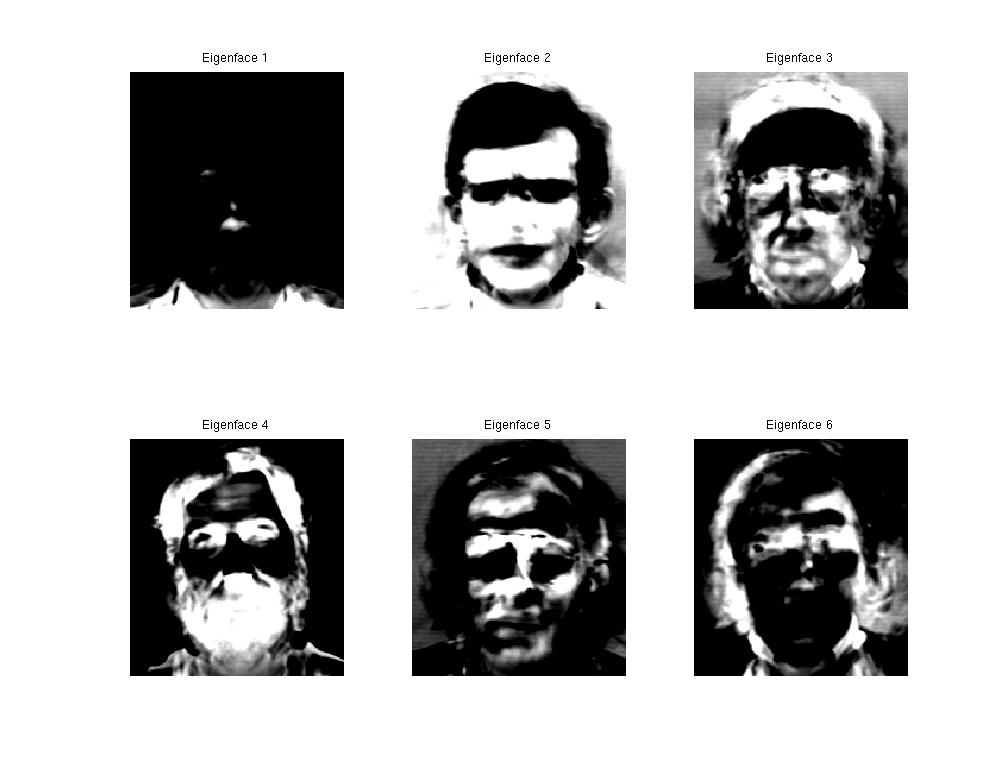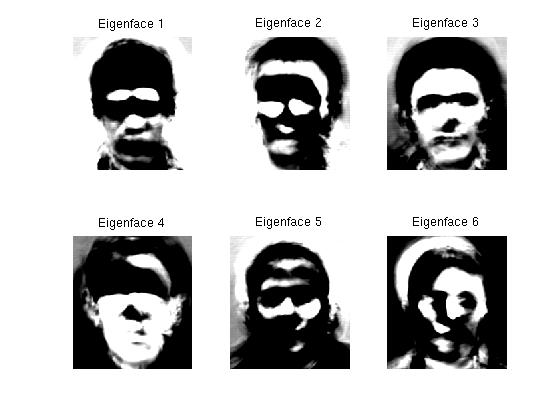# Face Recognition

## Alexandra Feldman (amf1) Final Project CS129 Spring 2011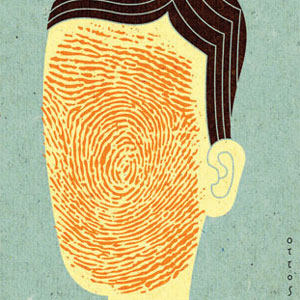### Applications of Face Recognition

As a computer vision problem, face recognition is one of the most sought-after unsolved problems in the industry. There are dozens of different implementations (a simple, albeit long, description of a lot of different approaches can be found here.

Face recognition is interesting as a computer vision problem, cognitive neuroscience problem, and in various industries. Areas that would benefit from robust face recognition include video surveillance, human-machine interaction, photo tagging, virtual reality, or law enforcement. Brown Cognitive Science classes teach that humans have special areas of the brain designated for face recognition, separate from other visual process. Others still think this is an open question.

The first face recognition engineers used semi-manual feature selection which computers could then use for recognition. This was in the early 1960's. Even today, the same problems with still plague even the best implementations: differences in posture, illumination, occlusion, age. You may be able to look at a picture of your grandmother on her wedding day and recognize her, but computers still can't.

### The Eigenface Implementation

The approach I decided to use was using eigenfaces and prinicple component analysis as the feature space for faces, and k-nearest neighbors for the machine learning classification part. I started with a large database of face images (courtesy of Dr. Libor Spacek). The theory behind eigenfaces is that using linear algebra techniques, we can represent the different "important features" of faces with the eigenvectors. Eigenfaces can be built for individual faces, or for a set of faces - I chose to do the latter.##### Figure 1: An example of the set of images available for one model.

Database details:
• 3 different directories (female, male, and male staff)
• 20-89 different people ("models") per directory
• 20 images per model - images taken within a few minutes, so variation between faces is small.
• Same background for each image
• = 2580 total images of faces.

Each face was converted to black and white to do the eigenface procedure.

### How to make eigenfaces: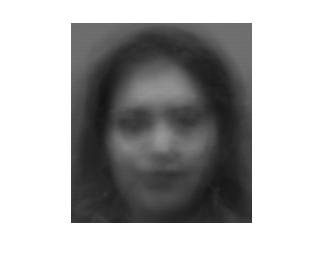##### Figure 2: M_avg: The mean of the female faces.
Given a set S of m images:

Create a matrix M where each row is an individual image (i.e. concatenate the rows of each image into a single row).

Compute the average face M_avg, and subtract that face from each row in M.

#### M = M - M_avg

Next, we would like to calculate the eigenvectors of the covariance matrix for each image, where the covariance matrix C is defined as:

#### C = (1/m) * SUM(Ai*Ai')

(A' is the transpose of matrix A)

Where Ai is the vectorized image from row i in M, and the SUM is over all images for i=[1,m]. So if the size of each image is n = height * width, then the size of the covariance matrix is n x n. Calculating the eigenvectors directly from this matrix is computationally infeasible, so instead I used this neat linear algebra trick (courtesy of Wikipedia):

Given the number of images m and mean-subtracted image matrix M,
we notice that if ui is an eigenvector M*M', then

#### vi = T' * ui

is an eigenvector of C. MM' is only an m x m matrix, so if then number of images you have is much smaller than the size of the images, this is a much faster way to compute the eigenvectors. In my case, running the face recognition algorithm on seperate image directories mean that n = 200 * 180 = 36,000; m <= 1780; so this trick is computationally advantageous.

We can get the eigenvectors of C and their associated eigenvalues using Matlab's handy eigenvector function eig:

#### T = M*M' [U,D] = eig(T)

(gets you a matrix U of ui eigenvectors and diagonal matrix D of their associated eigenvalues)

#### cov_eigenvectors = zeros(n,m) for i=1:m, u_i = U(:,i) v_i = M'*u_i cov_eigenvectors(:,i) = v_i end

(computes the eigenvectors of covariance matrix C)

Now we have the eigenvectors! Using Principle Component Analysis, the eigenvectors associated with the largest eigenvalues are the most significant representations of the face dataset. As the eigenvalues get smaller, the features in the corresponding eigenfaces get less discriminatory.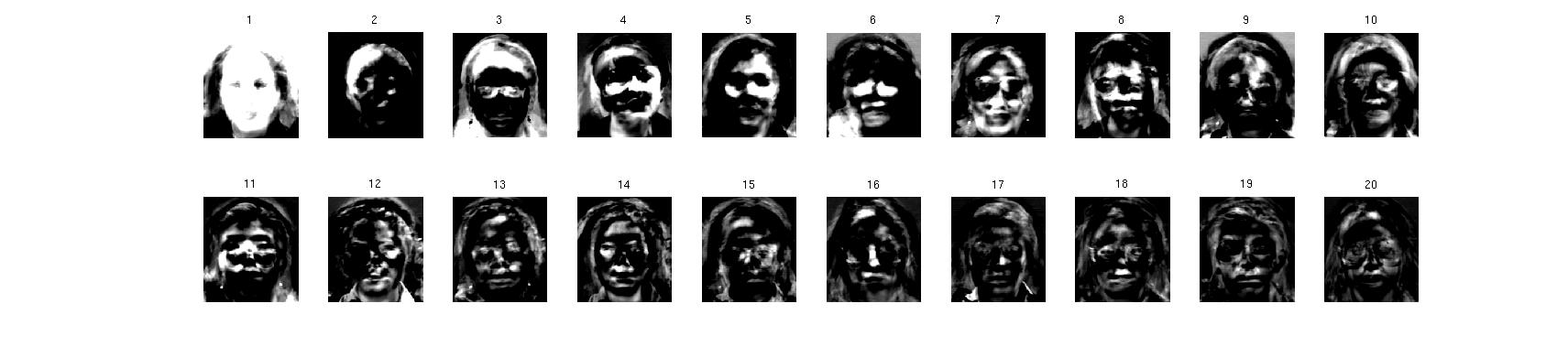##### Figure 3: The top 20 eigenfaces for the female database.
As we can see, the first several eigenfaces represent the most important "features" (white space): the face, hair, glasses, neck, cheekbones, etc. As the eigenvalues decrease, the eigenfaces become less binary, and more focused on smaller features. Some even look like they represent the face of a specific person as a feature, and not the cumulative features of the set as a whole. Theoretically, the complete set of canonical eigenfaces can be used to reconstruct any face using basic linear algebra; this set is just made from the available images, so it is catered to the faces we will be recognizing later.

### Machine learning on Eigenfaces: Training the classifier

I chose to use a kNN classifier to recognize faces. To do this, first train your classifier on the training data. Given the dataset described above, I randomly removed one image for each model and put it aside in a test set of images. The remaining 19 images per person were the training data. Using kNN requires that each training datum be represented as a point in d-dimensional space. In this case, d is however many of the principle components (eigenfaces) we want to use to construct each case. To avoid the curse of dimensionality, I set d = 36, which is smaller than the size of the training data set by orders of magnitude.

#### How to use eigenfaces to get points in 36-dimensional space:

Compute a difference matrix L for each of the m training images, where L is defined as a vector of scalars:

#### Li = ui * (Mi)

(recall: ui is the eigenface vector and Mi is L's mean-subtracted image vector)

This results in a vector of length 36, so L is the point in 36-dimensional space for a specific training image; the point is the image's numerical relation to the set of top eigenfaces. This is supervised learning, because for each point, we know which model it corresponds to, and can label (classify) each point accordingly.

### Using kNN to classify an image

Given a test image, compute the same matrix L as described above. Compute the Euclidean distance from this point to all the other points in the training data. For the top k points with smallest Euclidean distance, tally their votes: the majority vote is the resulting class we return for the test image.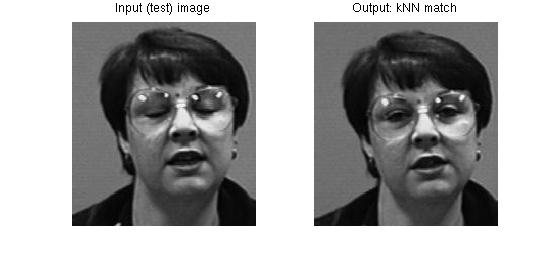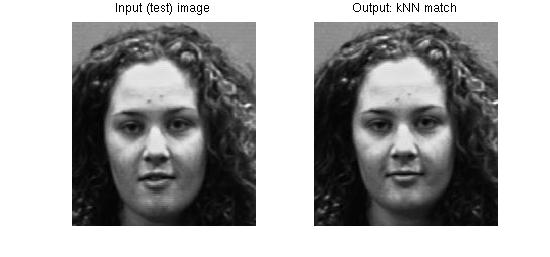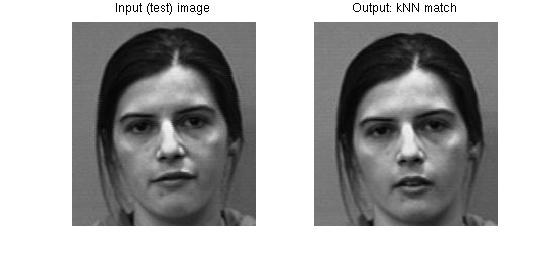### Results

It turns out that this approach works very well on small data sets (~20 models), but mediocrely on large (~100 models) datasets. For the small datasets (female and malestaff) it had a 100% detection rate; for the larger set (male) it was about 20%, which is much better than chance, but still not ideal.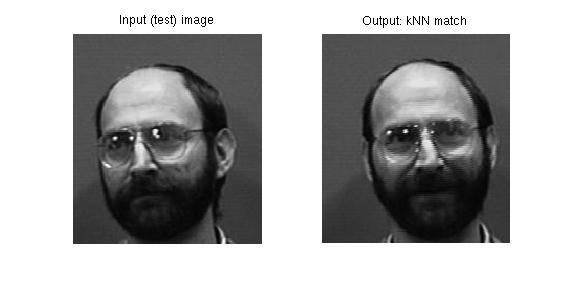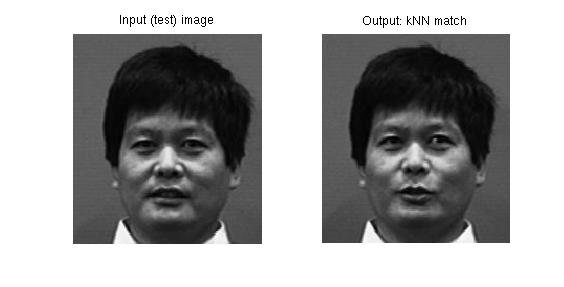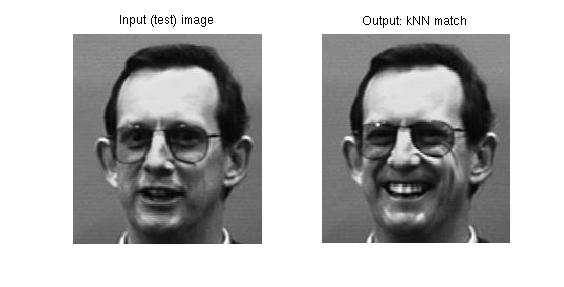#### Opportunities for Improvement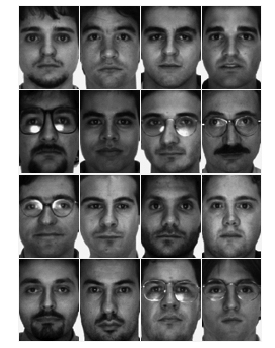Face DetectionRun the images through a face detection algorithm as preprocessing step (i.e. perform eigenface computation on a set of images that look more like the examples to the left, rather than figure 1). Using training data where the faces were more centralized would hopefully result in eigenfaces that defined actual facial features, and were more invariant to hair, background, pose, head size, etc.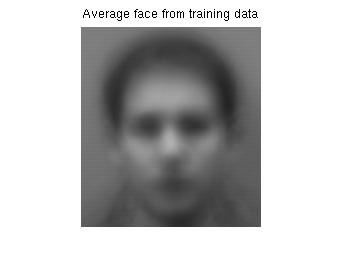Auto-alignmentAlign the images so that the eyes are in the same place in each figure as a preprocessing step; this would result in a more meaningful average face, and the resulting eigenfaces would be more representative of facial features rather than "image" features (i.e. if someone's head was more to the left of the picture frame rather than centered). The example on the right is an average face from Drexel's implementation of eigenfaces.The example on the left is my resulting mean face from the male directory.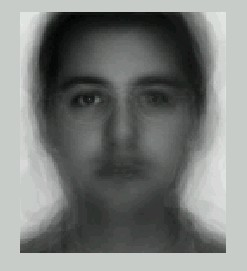Compute eigenfaces for each color channel instead of just black and white images Normalize gray space on faces to decrease illumination differences Tweak parameters (d, k, m) Add other features to feature space for kNN: e.g., distance between eyes, average skin color Use Hidden Markov Models to model faces instead of eigenfaces Use another classifier besides kNN (e.g. a support vector machine)

Eigenfaces for the male staff and male directories: Note that the most "significant" eigenface for the male staff is almost completely black; this could be improved upon if we made the changes to the training data described above.